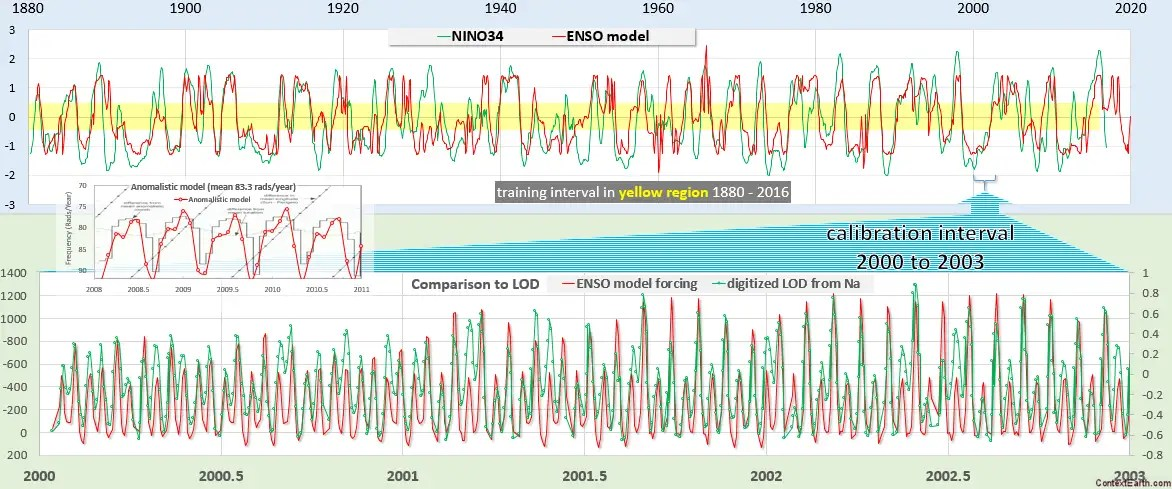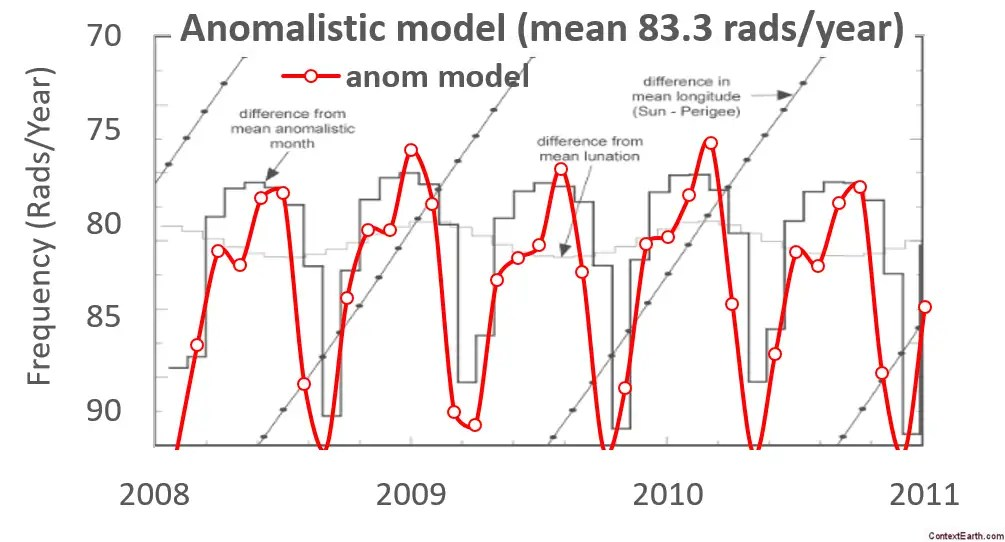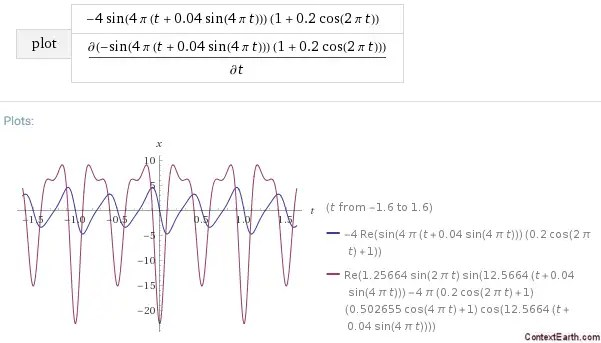# Variation in the Length of the Anomalistic Month

For the ENSO model, we use two constraints for the fitting process. One of the constraints is to maximize the correlation coefficient for the model over the ENSO training interval selected. The other constraint is to maximize the correlation of the selected lunar tidal forces over a measured Length-of-Day (LOD) interval. The latter constrains the lunar tidal forcing to known values that will actually change the angular momentum of the earth’s rotation. This in turn drives the sloshing of the Pacific ocean’s thermocline leading to the ENSO cycle. The two constraints are simultaneously met by heuristically maximizing the average of the correlation coefficients.

In addition, there are the fixed constraints of the primary lunar periods corresponding to the Draconic/nodal cycle and the Anomalistic cycle.

This combination gives a fairly effective fit over the entire training cycle, but there is an important additional constraint that needs to be applied to the Anomalistic cycle. The NASA eclispse and moon’s orbit page describes the situation :

“The anomalistic month is defined as the revolution of the Moon around its elliptical orbit as measured from perigee to perigee. The length of this period can vary by several days from its mean value of 27.55455 days (27d 13h 18m 33s). Figure 4-4 plots the difference of the anomalistic month from the mean value for the 3-year interval 2008 through 2010. … the eccentricity reaches a maximum when the major axis of the lunar orbit is pointed directly towards or directly away from the Sun (angles of 0° and 180°, respectively). This occurs at a mean interval of 205.9 days, which is somewhat longer than half a year because of the eastward shift of the major axis. “This is a significant variation in the anomalistic cycle over the course of a year. We don’t use this variation as a constraint but we can use it as a fitting parameter and then compare the variation obtained over that shown above.

Using the 205.9 day value ~365/(2-2/8.85), we break this into Fourier components of half this value and twice this value. The mean Anomalistic period of 27.5545 days is then frequency modulated by the slower periods by the standard engineering procedure. We then allow the amplitude and phase of each factor to vary during the training to obtain the best fit (this is slightly different than the concise form used previously).If we zoom in on the anomalistic period variation, we get this match to the NASA Goddard model:There is no reason to believe that this match would spontaneously occur given that there are 3 amplitudes and 3 phase factors involved. Yet it matches precisely to the (1) peak positions, (2) relative amplitudes, and to the (3) cusped shape via the Fourier series summation. An even better fit is obtained if we use abs(sin(π time/205.9+Φ)) as the fitting function as it naturally creates more of a cusp shape due to the full-wave rectification of the sine wave.

Conventional tidal analysis is renowned for being an exacting procedure , where the known tidal periods are broken down into equivalently similar sets of harmonic factors, yet applied on a diurnal or semidurnal basis. The only difference here is that ENSO responds to the monthly and fortnightly long-period tides and not the short-period ones.

→ This model fit gives further validation to the lunar tidal mechanism for forcing ENSO.  The exacting process of generating the correct lunar tidal variations (along with the subtle biennial modulation and the tricky aliasing) have likely contributed to the fact that the pattern has remained hidden for so long.  This is actually not so different a situation as the long hidden connection between triggering of earthquakes and the dynamic  moon-sun-earth alignment. That pattern is also hidden, only exposed recently. Alas, not everything can be quite as obvious as the pattern matching of ocean surface tides to the lunisolar cycles.

## References

 S. Consoli, D. R. Recupero, and V. Zavarella, “A survey on tidal analysis and forecasting methods for Tsunami detection,” arXiv preprint arXiv:1403.0135, 2014.

## UPDATE

The reason for the peculiar shape of the Anomalistic frequency variations is due to a different slope (i.e. velocity) on one lobe of the elliptical orbit than on the other. You can get this by generating another sinusoidal modulation on top of the average elliptical sinusoid. This generates an asymmetric sawtooth in the phase angle (see blue line below) and the characteristic spiked or cusped profile in the effective frequency or derivative of this value (see red line below).I am starting to use this formulation in the ENSO model as it is quite concise.

## One thought on “Variation in the Length of the Anomalistic Month”

1. Pingback: AMO | context/Earth